# Leray-Hirsch theorem for K-theory

## Statement

Let$p:E \to B$ be a fiber bundle with both$E$ and$B$ compact Hausdorff spaces and with fiber space$F$, such that :

•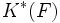$K^*(F)$ is free and finitely generated
• There exist classes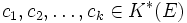$c_1, c_2, \ldots, c_k \in K^*(E)$ that restrict to a freely generating set for$K^*(F)$ for each fiber$F$

And suppose one of these conditions holds:

1.$B$ is a finite cell complex
2.$F$ is a finite cell complex having only cells of even dimension

Then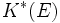$K^*(E)$ is free as a module over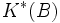$K^*(B)$, with basis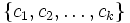$\{ c_1, c_2, \ldots, c_k \}$.